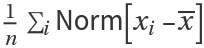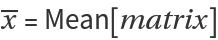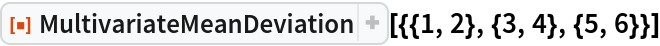Function Repository Resource:

# MultivariateMeanDeviation

Find the scalar mean of the Euclidean distances between the multivariate data points and their mean

Contributed by: Wolfram Research
 ResourceFunction["MultivariateMeanDeviation"][matrix] gives the mean of the Euclidean distances between the elements of matrix and their mean.

## Details and Options

ResourceFunction["MultivariateMeanDeviation"] is a univariate measure of mean deviation for multivariate data.
The multivariate mean deviation is given by, where matrix={x1,x2,,xn} and.
ResourceFunction["MultivariateMeanDeviation"] handles both numerical and symbolic data.

## Examples

### Basic Examples (1)

Multivariate mean deviation for bivariate data:

 In:=Out=## Requirements

Wolfram Language 11.3 (March 2018) or above

## Version History

• 1.0.0 – 20 February 2019